# Find the maximum numerical value in a list based on a criteria in Excel

To find the maximum numerical value in a list based on a criteria, we can use the MAX function along with the If function in Excel.

First we will learn how to use Max function in Microsoft Excel.

MAX: This function is used to return the largest number in a set of values. It ignores logical values and text.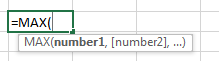Example: Range A1:A4containsnumbers, we need to return the maximum number from this range.

• Select the cell B1 and write the formula.
• =MAX(A1:A4), press Enter on your keyboard.
• The function will return 9.
• 9 is the maximum value in the range A1:A4.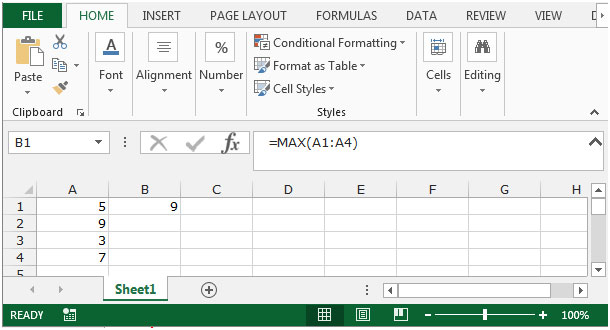Let’s take an example to understand how we can find the maximum numerical value in a list based on the criteria.

We have data for certain items in the range A2:D24. Column A contains items, Column B contains zone and column C contains sold unit.  We need to find the Maximum Units sold for each item zone-wise.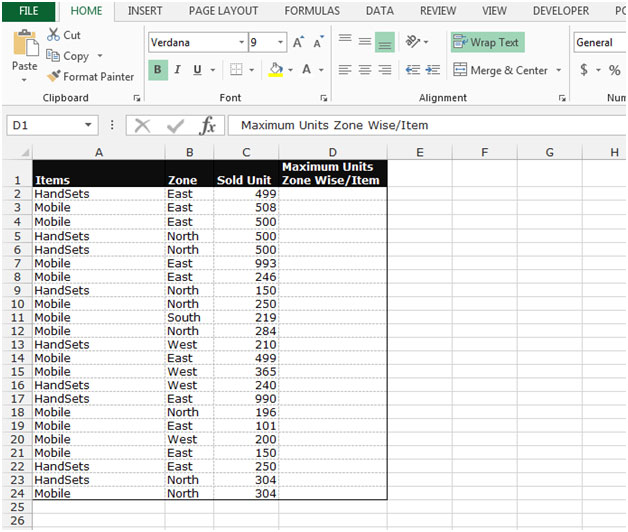• Select the cell D2.
• Write the formula to find out the maximum value
• =MAX((\$A\$2:\$A\$31=A2)*(\$B\$2:\$B\$31=B2)*(\$C\$2:\$C\$31))
• Press Ctrl+Shift+Enter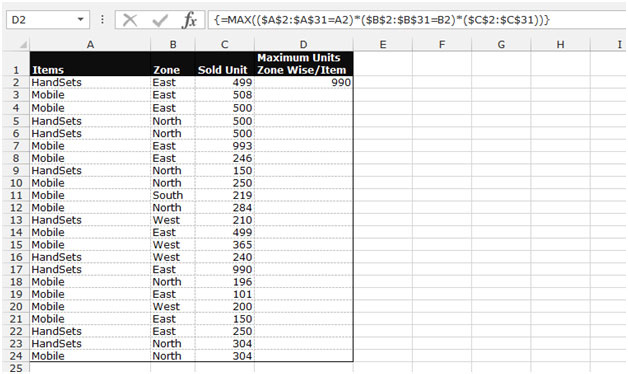• The function will return the maximum value in the east zone for handsets.
• By using this formula, you can find out the maximum value for each item zone-wise.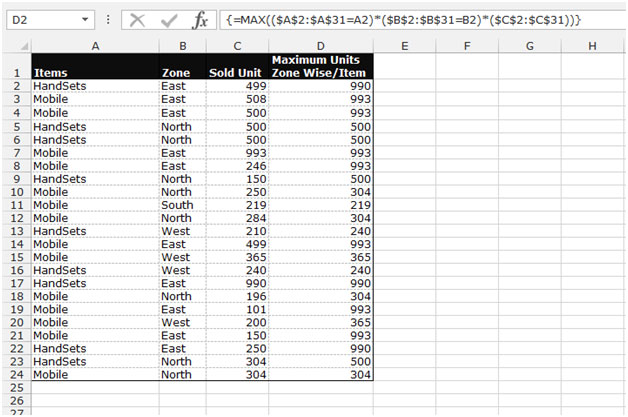Note:- This is an array function, so you cannot paste the formula in multiple cells. You have to paste the formula one by one in each cell.

This is the way we can find out the maximum number in the list based on criteria in Microsoft Excel.We would love to hear from you, do let us know how we can improve, complement or innovate our work and make it better for you. Write us at info@exceltip.com

## Users are saying about us...

1.Thank a lot.
Very Good Solution

2.I tried to use this with the =MIN() function and it did not work. I can get the maximum array function to work, but had to make sure that there weren't any blank rows. Does anyone know how to get the minimum value based on criteria from a separate column?

Terms and Conditions of use

The applications/code on this site are distributed as is and without warranties or liability. In no event shall the owner of the copyrights, or the authors of the applications/code be liable for any loss of profit, any problems or any damage resulting from the use or evaluation of the applications/code.